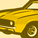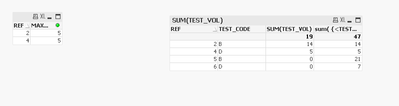QlikView App Dev

Discussion Board for collaboration related to QlikView App Development.

Announcements
QLIKWORLD LIVE! MAY 16 - 19TH, EARLY BIRD DISCOUNTS! REGISTER TODAY
cancel
Showing results for
Did you mean:Contributor III

Set Analysis help with p() function

Hello Experts,

I have this expression

sum(  {<TEST_CODE= p(MAX_Days)  >}      TEST_VOL  )  - the intend is to limit to all possible TEST_CODE values for the selection in MAX_Days. I am not sure whats wrong with this expression, but it returns 0

For testing, I've hard-coded a value and it works just fine.

sum(   {<    TEST_CODE= p( {<MAX_Days={10}> } )   >}     TEST_VOL)

Any idea?

Thanks,

Aji

Labels (2)

• Set Analysis

1 Solution

Accepted SolutionsPartner

Try this:

sum( {<TEST_CODE= p(TEST_CODE),MAX_Days>} TEST_VOL )

8 RepliesContributor III
Author

BTW the expression is

sum( {<TEST_CODE= p(MAX_Days), MAX_Days >} TEST_VOL )Partner

Does this expression:

sum( {< MAX_Days={"\$(=max(MAX_Days))"} >} TEST_VOL )Contributor III
Author

nope.. it doesn't workPartnerPartner

Try this:

sum( {<TEST_CODE= p(MAX_Days), TEST_CODE>} TEST_VOL )Contributor III
Author

Thanks for the suggestion .
Unfortunately this doesn't get what I am looking for
What I really need is, once the user make selection in MAX_Days, the expression calculates the TOT_VOL all the TEST_CODEs that has the selected MAX_days.

ex. if I select 5, it will limit to REF 2  and 4 in TABLE 2 ( blue color). Where as I want to find the SUM(TOT_VOL) for all B&D TEST_CODES ( Blue and Red)

TABLE 1

 REF MAX_Days 1 20 2 5 3 40 4 5 5 10 6 20

TABLE 2

 REF TEST_CODE TEST_VOL 1 A 5 1 A 6 1 A 7 1 A 8 2 B 6 2 B 8 3 C 3 3 C 4 3 C 5 4 D 3 4 D 2 5 B 20 5 B 1 6 D 1 6 D 4 6 D 2Partner

Try this:

sum( {<TEST_CODE= p(TEST_CODE),MAX_Days>} TEST_VOL )PartnerCommunity Browser